# Birth-and-death process

(diff) ← Older revision | Latest revision (diff) | Newer revision → (diff)

A Markov process with states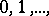in which in a time interval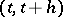transitions from the stateinto the statesand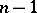occur with probabilitiesand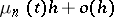, respectively, and where the probability of other transitions is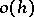. For a special choice of the reproduction coefficientsand the death coefficients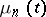one can obtain particular cases which provide a satisfactory description of various real processes: radioactive transformations, the running of telephone exchanges, the evolution of biological populations, etc. The fact that birth-and-death processes are widely used in applications is due to the simplicity of the equations for the transition probabilities, which often can be obtained explicitly. For instance, in the case of a Poisson process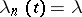,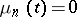, the probabilities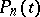(is the probability of being at timein the stateif the process has started from state) satisfy the system: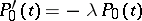whereThe solution of this system is: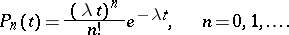A more general process is the one in which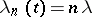,. This type of process was first studied by G. Yule (1924) in connection with the mathematical theory of evolution. A Yule process is a particular case of a pure birth process which is obtained from the general birth-and-death process by assuming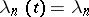,. If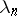increases very rapidly, then with positive probability one can pass through all states in a finite time and thenThe condition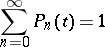for a pure birth process is satisfied if and only if the series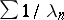diverges. If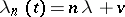,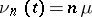, then the birth-and-death process is a branching process with immigration in which the statedesignates the number of particles. Each particle dies during timewith probability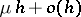, splits into two particles with probabilityand, moreover, one particle immigrates from the outside with probability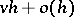. If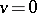then one obtains the simplest branching process without immigration. If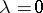and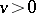, then this type of process with immigration can be applied to describe the functioning of a telephone exchange with an infinite number of lines. Here, the state is the number of occupied lines. The reproduction coefficientcharacterizes the rate of incoming telephone calls, andis the expected duration of a conversation.

How to Cite This Entry:
Birth-and-death process. Encyclopedia of Mathematics. URL: http://encyclopediaofmath.org/index.php?title=Birth-and-death_process&oldid=12092
This article was adapted from an original article by V.P. Chistyakov (originator), which appeared in Encyclopedia of Mathematics - ISBN 1402006098. See original article